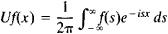# Unitary Operator

Also found in: Wikipedia.
The following article is from The Great Soviet Encyclopedia (1979). It might be outdated or ideologically biased.

## Unitary Operator

an extension of the notation of a rotation of Euclidean space to the infinite-dimensional case. More precisely, a unitary operator is a rotation of a Hilbert space about the origin. In analytic terms, an operator U that maps the Hilbert space H onto itself is said to be unitary if (f, g) = (Uf,Ug) for any two vectors f, g in H. A unitary operator preserves lengths of vectors and angles between vectors and is a linear operator. A unitary operator U has a unitary inverse U–1 such that U–1 = U*, where U* is the adjoint of U.

An example of a unitary operator is the Fourier-Plancherel operator, which associates to each function f(x), – ∞ < x < ∞, with square integrable absolute value the functionThe Great Soviet Encyclopedia, 3rd Edition (1970-1979). © 2010 The Gale Group, Inc. All rights reserved.
References in periodicals archive ?
proved that S([phi]([rho])) = S([rho]) ([for all][rho] [member of] S(H)) if and only if there exists a unitary operator U such that [phi]([rho]) = U[rho][U.sup.[dagger]], where [phi]([rho]) is a bistochastic quantum operation, i.e., [phi]([rho]) = [[summation].sub.i][E.sub.i][rho][E.sup.[dagger].sub.i] ([for all][rho] [member of] S(H)), and {[E.sub.i]} is a set of matrices known as operation elements.
Then [U.sub.p] is a unitary operator on [F.sup.2] and [U.sup.-1.sub.p] = [U.sub.-p] [10, Proposition 2.3].
The question of whether Austin Powder is an "operator" under the Mine Act came up in 15 dockets with 22 citations and orders that were consolidated at the Federal Mine Safety and Health Review Commission for the purpose of determining whether Austin Powder and its subsidiaries constitute a "unitary operator" for the purposes of the Mine Act.
exists for every unitary operator U [member of] B (H), then T is compact and l = 0 holds.
If we think of the measurement as dictated by Schroedinger's equation, then we should have a unitary operator [U.sub.M] [member of] H [cross product] [H.sub.I] with the following property:
Furthermore, because any unitary operator on d qubits can be factored into a product of two-level unitary operators on d qubits (Deutsch et al., 1995); and because these two-level unitary operators on d qubits can in turn be exactly (i.e.
Let J be a unitary operator in [L.sup.2]([??]) defined by
This is based on the Hadamard transformation, a unitary operator on [l.sub.2] ([SIGMA]) whose matrix with respect to the standard basis is
Quantum stochastic calculus was designed to describe the dynamics of quantum processes and we propose that we use it to study the non commutative Merton-Black-Scholes model in the following formulation (notice that our model includes also the Poisson process): We replace (see  for details on quantization) the stock process {[X.sub.T] / t [greater than or equal to] 0} of the classical Black-Scholes theory by the quantum mechanical process [j.sub.t](X) = [U.sup.*.sub.t] X [cross product] 1 [U.sub.t] where, for each t [greater than or equal to] 0, [U.sub.t] is a unitary operator defined on the tensor product H [cross product] [GAMMA]([L.sup.2]([R.sub.+], C)) of a system Hilbert space H and the noise Boson Fock space [GAMMA] = [GAMMA]([L.sup.2]([R.sub.+], C)) satisfying
Hence, A is a unitary operator, with D(A) = [L.sup.2.sub.2[pi]].
For each n [greater than or equal to] 0, there exists a unitary operator [U.sub.n] on [l.sup.2] (Z, H) such that
The question was posed and investigated in  and remains unanswered even if Banach-space power bounded operators are restricted to Hilbert-space contractions, where the problem is equivalently stated for unitary operators (see Remark 3 below), and also if weak supercyclicity is strengthened to weak l-sequential supercyclicity: does there exist a weakly unstable and weakly l-sequentially supercyclic unitary operator?

Site: Follow: Share:
Open / Close